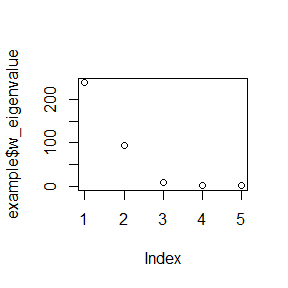# Introduction to BSSoverSpace

library(BSSoverSpace)
set.seed(16)

# Blind Source Separation Over Space

Blind Source Separation Over Space (BSSS) is a tool for analyzing spatial multivariate data. Blind Source Separation method assumes that observed variables are formed by linear combination of underlying independent latent variables, which cannot be observed directly. The goal is to estimate the latent variables, which also includes estimating the mixing matrix. This package BSSoverSpace is an implementation of the work Zhang, Hao, and Yao (2022). This manual provides introduction and simple instructions on how to use the functions within.

# The main function BSSS

In this package BSSoverSpace, the main function is BSSS. It implemented the method in Zhang, Hao, and Yao (2022) for estimating the latent field $$Z(s)$$, and the unmixing matrix. This function takes 5 inputs: x is the data matrix of observed random field $$X(s)$$. coord is the coordinate of observed random field $$X(s)$$. kernel_type and kernel_parameter are the specifications of kernels for us to select. For each kernel_type, specification of kernel_parameter slightly differs. If kernel_type equals 'ring', there must be an even number of parameters in kernel_parameter. Check spatial_kernel_matrix from package SpatialBSS for more details.

Here, we generate a random field and use it to demonstrate the usage of this function. First we generate 500 2-dimensional coordinates:

sample_size <- 500
coords <- runif(sample_size * 2) * 50
dim(coords) <- c(sample_size, 2)

Next, we generate a 5-variate latent gaussian random field $$Z(s)$$ with matern covariance function in the following way:

library('BSSoverSpace')
dim <- 5 # specify the dimensionality of random variable
nu <- runif(dim, 0, 6) # parameter for matern covariance function
kappa <- runif(dim, 0, 2) # parameter for matern covariance function
zs <- gen_matern_gaussian_rf(coords=coords, dim=dim, nu=nu, kappa=kappa)

Then, we create a mixing matrix $$\Omega$$ , and mix our latent field to get the observed random field $$X(s)$$:

mix_mat <- diag(dim) # create a diagonal matrix as the mixing matrix
xs <- t(mix_mat %*% t(zs))

Now the observed random field $$X(s)$$ is created, and we need to choose kernels. Here we choose 3 ring kernels, with parameters $$(0, 0.5)$$, $$(0.5, 1)$$ and $$(1, 8)$$.

example<-BSSS(xs, coord = coords, kernel_type = 'ring', kernel_parameter = c(0, 0.5, 0.5, 1, 1, 8))

The function BSSS returns both the estimated mixing matrix $$\hat{\Omega}$$ mix_mat_est and the estimated latent field $$\widehat{Z(s)}$$. To see how good $$\hat{\Omega}$$ is, we can use function d_score, which gives a numeric value between 0 and 1, with 0 meaning that the estimator is a column permutation of true value:

d_score(example$mix_mat_est, mix_mat) #>  0.123749 We can further explore the validity of our estimation, by looking at the eigenvalues of $$\hat{W}$$. Larger gap between first few eigenvalues would strengthen the validity of our estimation. One can see the details of $$\hat{W}$$ in Zhang, Hao, and Yao (2022). plot(example$w_eigenvalue)